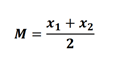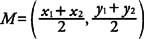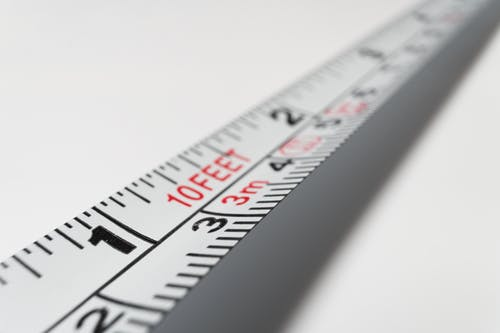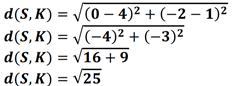# Teaching Midpoint and Distance in the Coordinate Plane

When learning about midpoint and distance in the coordinate plane, children learn how to apply the formula for finding the midpoint, as well as use the distance formula and the Pythagorean theorem to find the distance between two points.

As a teacher, you may find that, sometimes, children struggle with these math concepts, and you may be wondering what are the best ways to support them. To help out, we’ve created a list of guidelines that you can follow to keep your students engaged throughout the lesson.

## Midpoint Definition

Explain to students that a midpoint of a segment is simply a point that divides the segment into two equal segments.

Draw a number line on the whiteboard to demonstrate this or simply print out this Guided Notes Worksheet (Members Only) and distribute it to students.### The Midpoint Formula

Explain that to find the coordinates of a midpoint of a segment on a number line, when the endpoints of the midpoint have coordinates x1 and x2 we use the following formula:You can illustrate the application of the formula with an example, such as:

Find the coordinates of the midpoint of the segment with these endpoints: x1 = -5 and x2 = 1.

– 5+1
M =  ___
2

-4
M = __
2

M = – 2Draw a number line and present this on the number line so that students can see that the midpoint is indeed -2.

Now remind children what a coordinate plane is. It is simply a plane that is divided into four equal sections, also known as quadrants, by the intersection of a vertical line (y-axis) and a horizontal line (x-axis).

The coordinates, or simply location, of a point in a coordinate plane, are provided through its ordered pair, that is, (x, y). Explain that to find the midpoint on the coordinate plane, we use the following midpoint formula:Use the formula in an example, such as:

Find the coordinates of the midpoint on a segment CD with the following endpoints C (6, -1) and D (4, 2).

Explain that first, we’ll write down the x and y values, knowing that x always comes before y. In other words, in terms of endpoint C (6, -1), we can infer that x1 = 6 and y1 = – 1, whereas for endpoint D (4, 2), we can see that x2 = 4 and y2 = 2.

By replacing these values in our formula, we’ll get:Draw a coordinate plane to help children visualize that the coordinates of the midpoint of the segment CD are (5, ½).

## Distance in the Coordinate Plane Definition

Explain that when we talk about the distance on the coordinate plane, we’re simply referring to the distance between two points on the coordinate plane, such as point A (x1, y1) and point B (x2, y2).

Point out that the symbol for distance is the letter d.### Distance Formula

Explain how to calculate the distance between two points on the coordinate plane, A (x1, y1) and B (x2, y2), we use the following formula:Provide an example of how we use the distance formula, such as:

Find the distance between these two points S (4, 1) and K (0, -2). d (S, K) = ?

By applying the above formula, we’ll get:So d (S, K) = 5, or the distance between S and K is 5.

If you have a membership for MathTeacherCoach, use this Guided Notes Worksheet (Members Only) to provide additional guidelines, as well as examples of how formulas for finding the midpoint and distance in the coordinate plane are used.

## Activities to Practice Midpoint and Distance in the Coordinate Plane

### Distance Formula Sum Game

This is a simple game that can be played in any classroom setting. Use this Assignment Worksheet (Members Only), as well as this Exit Quiz Worksheet (Members Only) to create math cards. On each card, write one math problem related to distance in the coordinate plane.

Divide students into groups of four and provide one card per student. Each student should try to solve the math problem. Once all group members have figured out the answer to their cards, they should add each of their numbers to form a ‘group sum’.

They then consult the teacher if their group sum is correct. If it isn’t, they have to review each math card together. In this sense, this game is great for fostering peer tutoring and cooperation among students.

The first group to have a correct sum wins the game!

### Midpoint Online GameUse this online game by IXL to further practice finding the midpoint in the coordinate plane by using the midpoint formula. Provide a suitable device for each student and start playing!

Students are presented with diverse math problems, which they have to work out on their own.

Since they often have to graph the math problems on the coordinate plane, students tend to enjoy this game, especially if playing on a device with a touchpad.

If they make a mistake, the game provides the correct answer, alongside an elaborate explanation of why this is the correct answer. Bearing this in mind, the game is great for homeschooling parents as well.

## Before You Leave…

If you enjoyed our guidelines on teaching midpoint and distance in the coordinate plane, you’ll love our full lesson and worksheets on this topic – so why not sign up for our emails to receive loads of free content!

You can also join us in the MathTeacherCoach community or simply head over to our blog, where you’ll find a variety of math resources and worksheets for children of all ages!